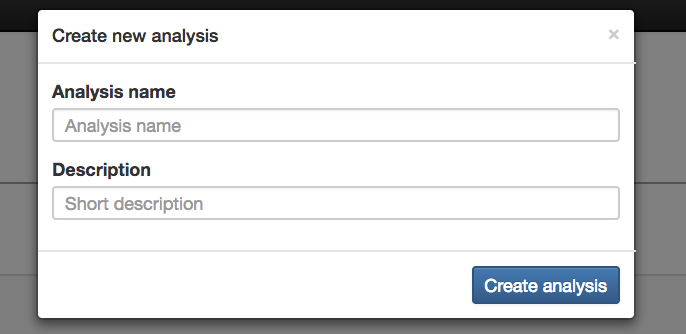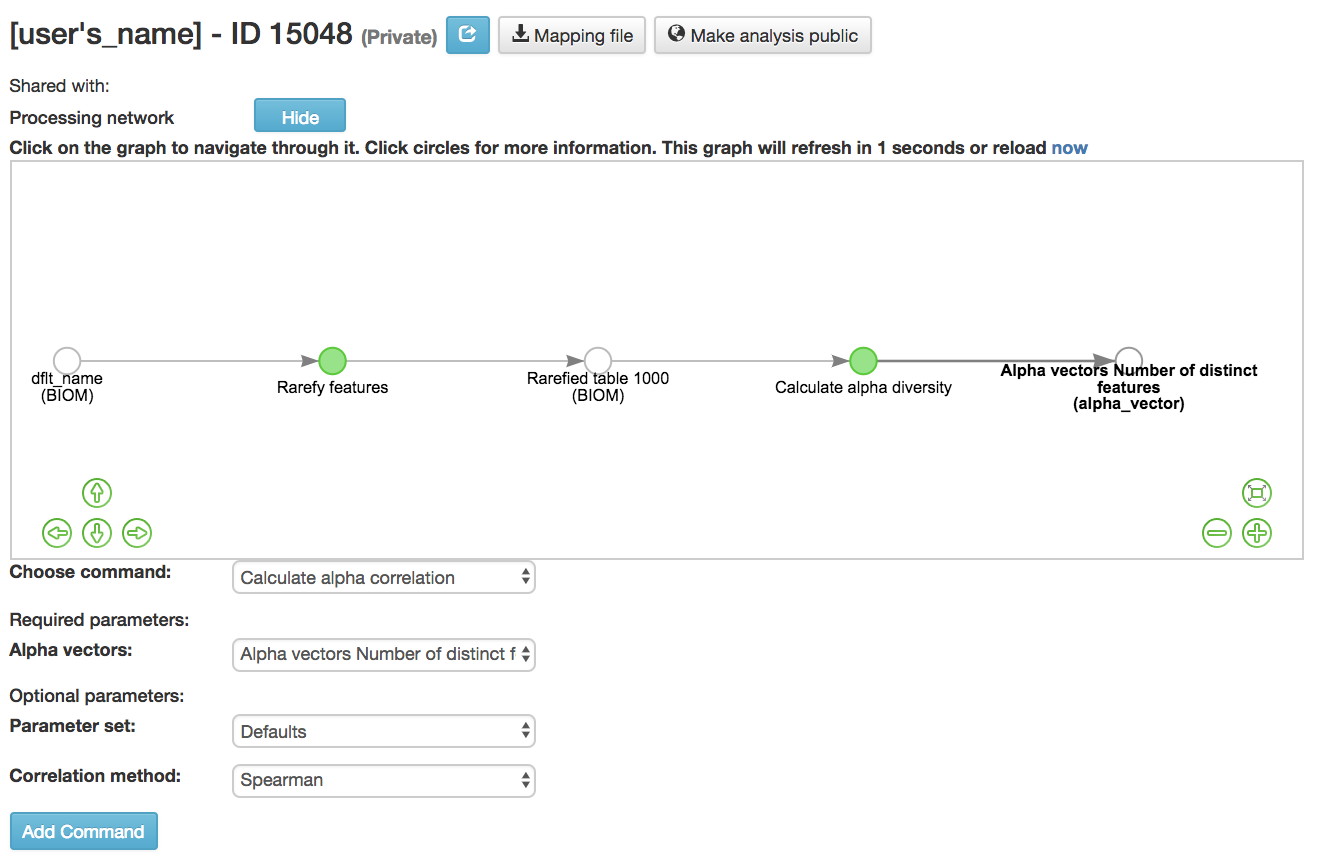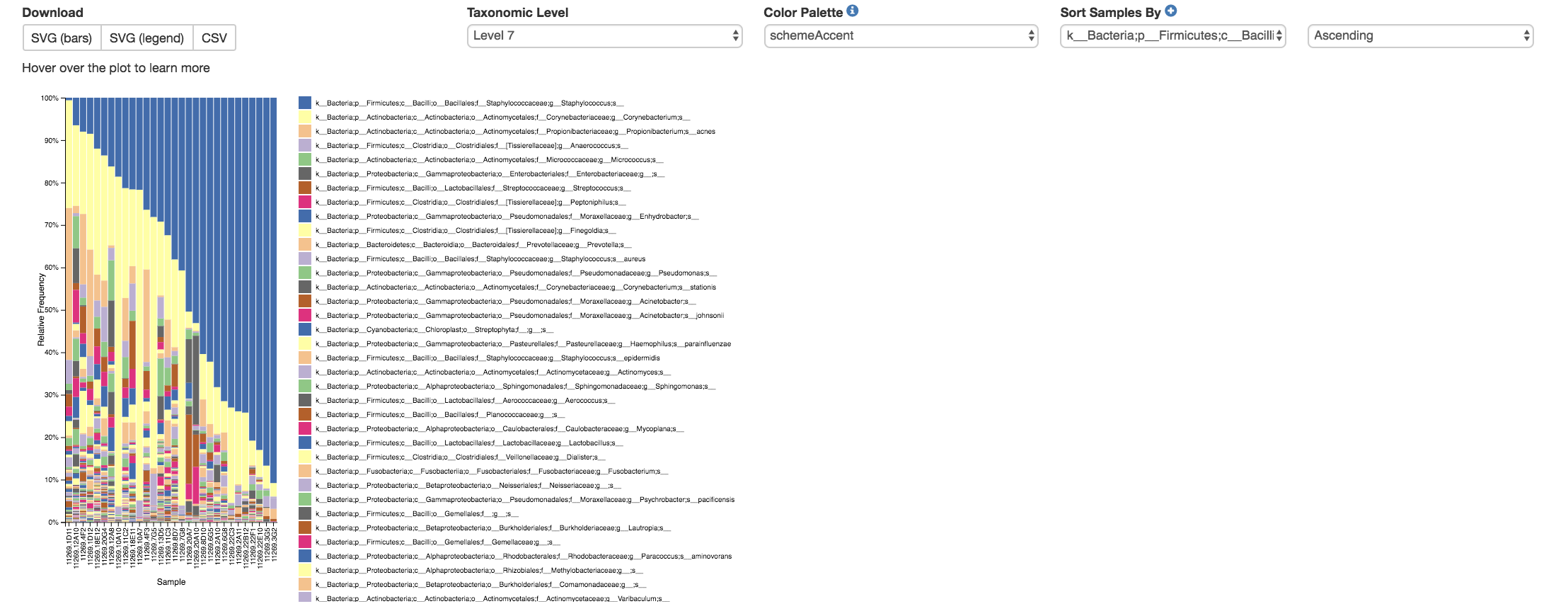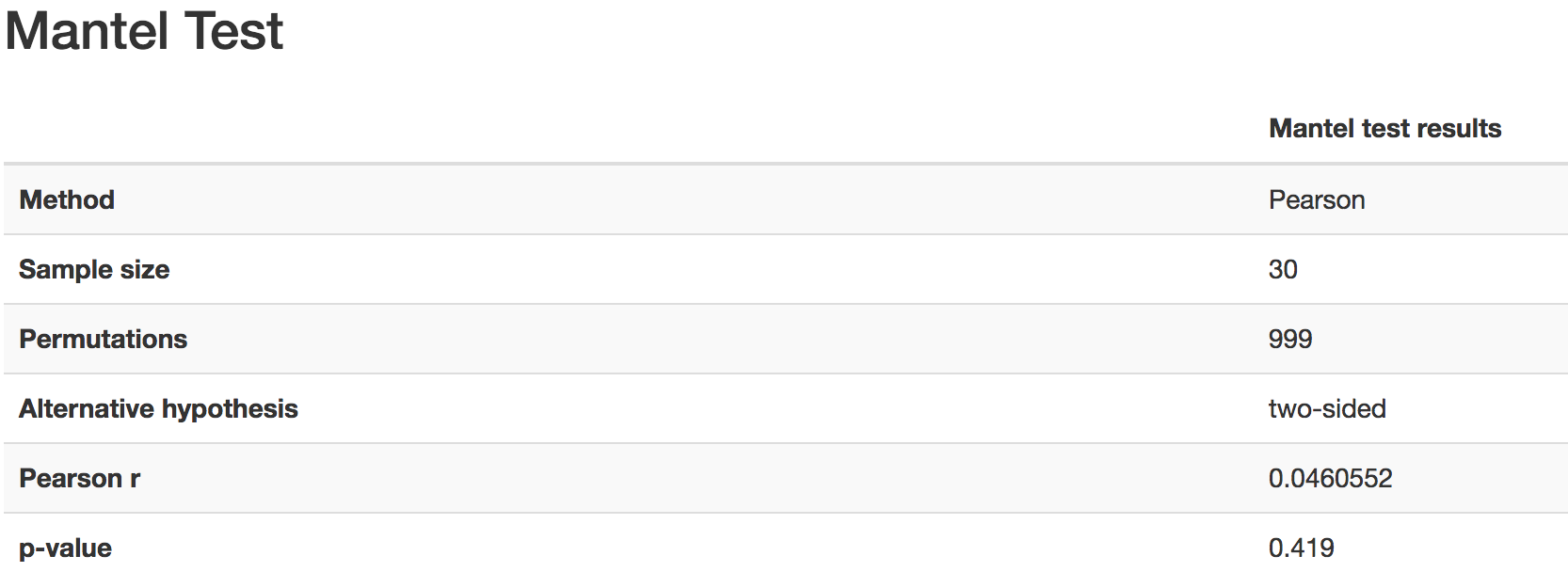# Analyzing Samples¶

## Qiita now uses QIIME2 plugins for analysis.¶

Thanks to this, we’ve got new layout of the analysis panel and the following new features:

## Creating A New Analysis¶• Create New Analysis Page

• Filter results by column data (Title, Abstract, PI, etc.): Searches for studies with the title/abstract/PI/etc. that you inputted

• Filter study by Study Tags: Searches for studies with the tag you searched for

• Title: Brings you to Study Information Page of that experiment

• Green Expand for Analysis Button: Reveals the studies done on this data that can be used for further analysis

• Per Artifact Button: Reveals the names of the artifacts, the number of samples in the prep info, and the files

• More than 1 can be done at once to do large meta-data analysis• Create New Analysis: Creates the analysis using the data that has been added

• Analysis Name (required): Name for the analysis that will be done

• Description (optional): Description for the analysis that will be done

## Single vs. Meta Analysis¶

• Single analysis: One study chosen to analyze

• Meta-analysis: Multiple studies chosen to analyze

• You can only merge like data

## Processing Network Page: Commands¶

### Rarefying Features¶• Rarefy features: Subsample frequencies from all samples without replacement so that the sum of frequencies in each sample is equal to the sampling-depth.

• BIOM table (required): Feature table containing the samples for which features should be rarefied

• Parameter set: Parameters at which the rarefication is run

• Sampling depth (required): Total frequency that each sample should be rarefied to, samples where sum of frequencies is less than sampling depth will not be included in resulting table

Note that rarefaction has some advantages for beta-diversity analyses , but can have undesirable properties in tests of differential abundance . To analyze your data with alternative normalization strategies, you can easily download the raw biom tables (see Downloading From Qiita) and load them into an analysis pipeline such as Phyloseq.• Filter samples by metadata: Filters samples from an OTU table on the basis of the number of observations in that sample, or on the basis of sample metadata

• BIOM table (required): Feature table containing the samples for which features should be filtered

• Maximum feature frequency across samples (optional): Maximum total frequency that a feature can have to be retained

• Maximum features per sample (optional): Maximum number of features that a sample can have to be retained

• Minimum feature frequency across samples (optional): Minimum total frequency that a feature must have to be retained

• Minimum features per sample (optional): Minimum number of features that a sample can have to be retained

• SQLite WHERE-clause (optional): Metadata group that is being filtered out

• If you want to filter your samples by body_site and you want to only keep the tongue samples, fill the clause this way: `body_site = 'UBERON:tongue'`

• If you want to filter your samples by body_site and you want to only remove the tongue samples, fill the clause this way: `body_site != 'UBERON:tongue'`

### Summarizing Taxa¶• Summarize Taxa: Creates a bar plot of the taxa within the analysis

• Can only be performed with closed-reference data

• BIOM table (required): Feature table containing the samples to visualize at various taxonomic levels

### Calculating Alpha Diversity¶• Calculate alpha diversity  : Measures the diversity within a sample

• BIOM table (required): Feature table containing the samples for which alpha diversity should be computed

• Diversity metric (required): Alpha diversity metric to be run

• Abundance-based Coverage Estimator (ACE) metric  : Calculates the ACE metric

• Estimates species richness using a correction factor

• Berger-Parker Dominance Index  : Calculates Berger-Parker dominance index

• Relative richness of the abundant species

• Brillouin’s index  : Calculates Brillouin’s index

• Measures the diversity of the species present

• Use when randomness can’t be guaranteed

• Chao1 index  : Calculates Chao1 index

• Estimates diversity from abundant data

• Estimates number of rare taxa missed from undersampling

• Dominance measure: Calculates dominance measure

• How equally the taxa are presented

• Effective Number of Species (ENS)/Probability of intra-or interspecific encounter (PIE) metric  : Calculates Effective Number of Species (ENS)/Probability of intra-or interspecific encounter (PIE) metric

• Shows how absolute amount of species, relative abundances of species, and their intraspecific clustering affect differences in biodiversity among communities

• Faith’s phylogenetic diversity  : Calculates faith’s phylogenetic diversity

• Measures of biodiversity that incorporates phylogenetic difference between species

• Sum of length of branches

• Fisher’s index  : Calculates Fisher’s index

• Relationship between the number of species and the abundance of each species

• Gini index  : Calculates Gini index

• Measures species abundance

• Assumes that the sampling is accurate and that additional data would fall on linear gradients between the values of the given data

• Good’s coverage of counts  : Calculates Good’s coverage of counts.

• Estimates the percent of an entire species that is represented in a sample

• Heip’s evenness measure  : Calculates Heip’s evenness measure.

• Removes dependency on species number

• Estimates how much of the environment contains unsampled taxa

• Best estimate on a complete sample

• Margalef’s richness index  : Calculates Margalef’s richness index

• Measures species richness in a given area or community

• Mcintosh dominance index D  : Calculates McIntosh dominance index D

• Affected by the variation in dominant taxa and less affected by the variation in less abundant or rare taxa

• Mcintosh evenness index E  : Calculates McIntosh’s evenness measure E

• How evenly abundant taxa are

• Menhinick’s richness index  : Calculates Menhinick’s richness index

• The ratio of the number of taxa to the square root of the sample size

• Michaelis-Menten fit to rarefaction curve of observed OTUs  : Calculates Michaelis-Menten fit to rarefaction curve of observed OTUs.

• Estimated richness of species pools

• Number of distinct features  : Calculates number of distinct OTUs

• Number of double occurrences: Calculates number of double occurrence OTUs (doubletons)

• OTUs that only occur twice

• Number of single occurrences: Calculates number of single occurrence OTUs (singletons)

• OTUs that appear only once in a given sample

• Pielou’s evenness  : Calculates Pielou’s eveness

• Measure of relative evenness of species richness

• Robbins’ estimator  : Calculates Robbins’ estimator

• Probability of unobserved outcomes

• Shannon’s index  : Calculates Shannon’s index

• Calculates richness and diversity using a natural logarithm

• Accounts for both abundance and evenness of the taxa present

• Simpson evenness measure E  : Calculates Simpson’s evenness measure E.

• Diversity that account for the number of organisms and number of species

• Simpson’s index  : Calculates Simpson’s index

• Measures the relative abundance of the different species making up the sample richness

• Strong’s dominance index (Dw)  : Calculates Strong’s dominance index

• Measures species abundance unevenness

• Phylogenetic tree (required for Faith PD): Phylogenetic tree to be used with alpha analyses (only include when necessary)

• Currently the only tree that can be used is the GreenGenes 97% OTU based phylogenetic tree

### Calculating Beta Diversity¶• Calculate beta diversity  : Measured the diversity between samples

• BIOM table (required): Feature table containing the samples for which beta diversity should be computed

• Weighs distances based on the proportion of the relative abundance represented between the samples at a given node under evaluation

• Alpha value (Generalized UniFrac only): Controls importance of sample proportions

• 1.0 is weighted normalized UniFrac. 0.0 is close to unweighted UniFrac, but only if the sample are dichotomized.

• Bypass tips (phylogenetic only): In a bifurcating tree, the tips make up about 50% of the nodes in a tree. By ignoring them, specificity can be traded for reduced compute time. This has the effect of collapsing the phylogeny, and is analogous (in concept) to moving from 99% to 97% OTUs

• Diversity metric (required): Beta diversity metric to be run

• Bray-Curtis dissimilarity  : Calculates Bray–Curtis dissimilarity

• Fraction of overabundant counts

• Canberra distance  : Calculates Canberra distance

• Overabundance on a feature by feature basis

• Chebyshev distance  : Calculates Chebyshev distance

• Maximum distance between two samples

• City-block distance  : Calculates City-block distance

• Similar to the Euclidean distance but the effect of a large difference in a single dimension is reduced

• Correlation coefficient  : Measures Correlation coefficient

• Measure of strength and direction of linear relationship between samples

• Cosine Similarity  : Measures Cosine similarity

• Ratio of the amount of common species in a sample to the mean of the two samples

• Dice measures  : Calculates Dice measure

• Statistic used for comparing the similarity of two samples

• Only counts true positives once

• Euclidean distance  : Measures Euclidean distance

• Species-by-species distance matrix

• Generalized Unifrac  : Measures Generalized UniFrac

• Detects a wider range of biological changes compared to unweighted and weighted UniFrac

• Hamming distance  : Measures Hamming distance

• Minimum number of substitutions required to change one group to the other

• Jaccard similarity index  : Calculates Jaccard similarity index

• Fraction of unique features, regardless of abundance

• Kulczynski dissimilarity index  : Measures Kulczynski dissimilarity index

• Describes the dissimilarity between two samples

• Matching components  : Measures Matching components

• Compares indices under all possible situations

• Rogers-tanimoto distance  : Measures Rogers-Tanimoto distance

• Allows the possibility of two samples, which are quite different from each other, to both be similar to a third

• Russel-Rao coefficient  : Calculates Russell-Rao coefficients

• Equal weight is given to matches and non-matches

• Sokal-Michener coefficient  : Measures Sokal-Michener coefficient

• Proportion of matches between samples

• Sokal-Sneath Index  : Calculates Sokal-Sneath index

• Measure of species turnover

• Species-by-species Euclidean  : Measures Species-by-species Euclidean

• Standardized Euclidean distance between two groups

• Each coordinate difference between observations is scaled by dividing by the corresponding element of the standard deviation

• Squared Euclidean  : Measures squared Euclidean distance

• Place progressively greater weight on samples that are farther apart

• Unweighted Unifrac  : Measures unweighted UniFrac

• Measures the fraction of unique branch length

• Weighted Minkowski metric  : Measures Weighted Minkowski metric

• Allows the use of the k-means-type paradigm to cluster large data sets

• Weighted normalized UniFrac  : Measures Weighted normalized UniFrac

• Takes into account abundance

• Normalization adjusts for varying root-to-tip distances.

• Weighted unnormalized UniFrac  : Measures Weighted unnormalized UniFrac

• Takes into account abundance

• Doesn’t correct for unequal sampling effort or different evolutionary rates between taxa

• Yule index  : Measures Yule index

• Measures biodiversity

• Determined by the diversity of species and the proportions between the abundance of those species.

• Number of jobs: Number of workers to use

• Phylogenetic tree (required for Weighted Minkowski metric and all UniFrac metrics): Phylogenetic tree to be used with beta analyses (only include when necessary)

• Currently the only tree that can be used is the GreenGenes 97% OTU based phylogenetic tree

### Calculating Alpha Correlation¶• Calculate alpha correlation  : Determines if the numeric sample metadata category is correlated with alpha diversity

• Alpha Vectors (required): Vector of alpha diversity values by sample

• Correlation Method (required): Correction test being applied

• Spearman  : Measures if there is a linear relationship between 2 variables

• Pearson  : Measures how strong the linear relationship is between 2 variables

### Performing Principal Coordinate Analysis¶• Perform Principal Coordinate Analysis (PCoA)  : Visualizes the similarities and differences between samples using Emperor Plots 

• Distance matrix (required): Distance matrix on which the PCoA should be computed

### Calculating Beta Group Significance¶• Calculate beta group significance: Determines whether groups of samples are significantly different from one another using a permutation-based statistical test

• Distance matrix (required): Matrix of distances between pairs of samples

• Comparison Type (required): Perform or not perform pairwise tests between all pairs of groups in addition to the test across all groups

• Method (required): Correlation test being applied

• Anosim  : Describes the strength and significance that a category has in determining the distances between points and can accept either categorical or continuous variables in the metadata mapping file

• Permanova  : Describes the strength and significance that a category has in determining the distances between points and can accept categorical variables

• Number of permutations (required): Number of permutations to be run when computing p-values

### Calculating Beta Correlation¶• Calculate beta correlation: Identifies a correlation between the distance matrix and a numeric sample metadata category

• Distance-matrix (required): Matrix of distances between pairs of samples

• Correlation method (required): Correlation test being applied

• Spearman  : Measures if there is a linear relationship between 2 variables

• Pearson  : Measures how strong the linear relationship is between 2 variables

• Number of permutations (required): Number of permutations to be run when computing p-values

## Processing Network Page: Results¶

### Taxa Bar Plot¶• Taxonomic Level: How specific the taxa will be displayed

• 1- Kingdom, 2- Phylum, 3- Class, 4- Order, 5- Family, 6- Genus, 7- Species

• Color Palette: Changes the coloring of your taxa bar plot

• Discrete: Each taxon is a different color

• Continuous: Each taxon is a different shade of one color

• Sort Sample By: Sorts data by sample metadata or taxonomic abundance and either by ascending or descending order

### Alpha Diversity Box Plots and Statistics¶• Boxplot: Shows how different measures of alpha diversity correlate with different metadata categories

• Category: Choose the metadata category you would like to analyze

• Kruskal-Wallis  : Result of Kruskal-Wallis tests

• Says if the differences are statistically significant

### Alpha Correlation Box Plots and Statistics¶• Boxplot: Shows how different measures of alpha diversity correlate with different metadata categories

• Gives the Spearman or Pearson result (rho and p-value)

### Beta Diversity Distance Matrix¶• Distance Matrix: Dissimilarity value for each pairwise comparison

### Principal Coordinate Analysis Plot¶• Emperor Plot: Visualization of similarities/dissimilarities between samples

• Color: Choose colors for each group

• Color Category: Groups each sample by the given category chosen by a given color

• Visibility Allows for making certain samples invisible

• Does not remove them from the analysis

• Must perform filtering to do that

• Opacity: Change the transparency of a given category

• Scale: Change the size of a given category

• Shape: Groups each sample by the given category chosen by a given shape

• Axes: Change the position of the axis as well as the color of the graph

• Animations: Traces the samples sorted by a metadata category

• Requires a gradient column (the order in which samples are connected together, must be numeric) and a trajectory column (the way in which samples are grouped together) within the sample information file

• Works best for time series

### Beta Group Significance Box Plots and Statistics¶• Boxplot: Shows how different measures of beta diversity correlate with different metadata categories

• Gives the Permanova or Anosim result (psuedo-F and p-value)

### Beta Correlation¶• Gives the Spearman or Pearson result (rho and p-value)

• Spearman  : Measures if there is a linear relationship between 2 variables

• Pearson  : Measures how strong the linear relationship is between 2 variables

• Gives scatterplot of the distance matrix on the x-axis and the variable being tested on the y-axis